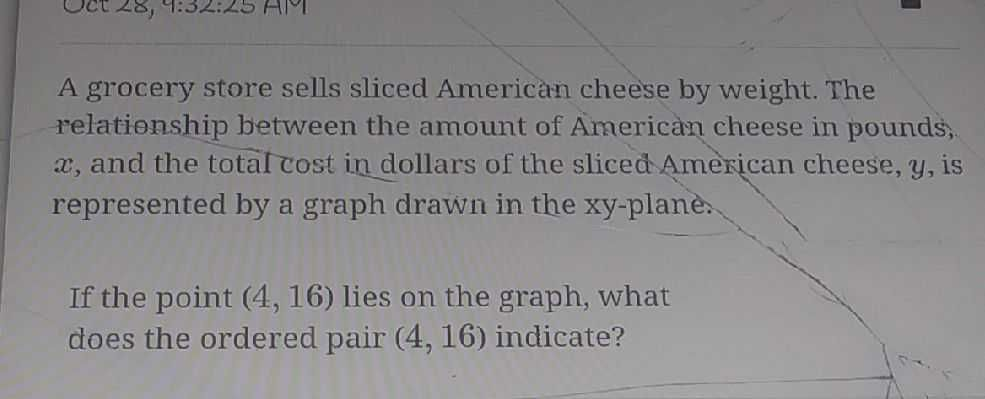### ¿Todavía tienes preguntas de matemáticas?

Pregunte a nuestros tutores expertos
Algebra
PreguntaA grocery store sells sliced American cheese by weight. The relationship between the amount of American cheese in pounds,

$$x$$ , and the total cost in dollars of the sliced American cheese, $$y$$ , is represented by a graph drawn in the xy-plane. If the point $$( 4,16 )$$ lies on the graph, what does the ordered pair $$( 4,16 )$$ indicate?

The total cost of 4 slices of cheese is $$\$$16.